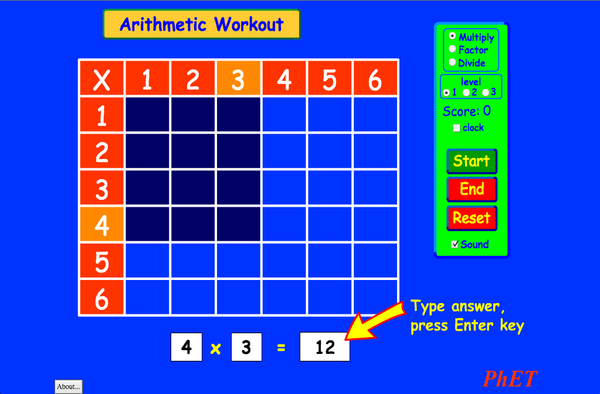# 算數下載嵌入 關閉 嵌入模擬教學執行複本 利用 HTML 來嵌入模擬教學執行複本。您可以在 HTML 中改變已嵌入模擬教學的寬度及高度。 嵌入啓動模擬教學之圖樣返回 HTML5 版本

### 標題

• Multiplication

HTML5 版本之翻譯：

### 學習目標

• ●用乘法表幫助了解乘法、除法和商
• ●增加乘法、除法和商的準確度
• ●發展計算能力

### 標準對齊

#### 共用核心 - 數學

3.OA.A.1
Interpret products of whole numbers, e.g., interpret 5 × 7 as the total number of objects in 5 groups of 7 objects each. For example, describe a context in which a total number of objects can be expressed as 5 × 7.
3.OA.A.2
Interpret whole-number quotients of whole numbers, e.g., interpret 56 ÷ 8 as the number of objects in each share when 56 objects are partitioned equally into 8 shares, or as a number of shares when 56 objects are partitioned into equal shares of 8 objects each. For example, describe a context in which a number of shares or a number of groups can be expressed as 56 ÷ 8.
3.OA.A.4
Determine the unknown whole number in a multiplication or division equation relating three whole numbers. For example, determine the unknown number that makes the equation true in each of the equations 8 × ? = 48, 5 = _ ÷ 3, 6 × 6 = ?
3.OA.B.5
Apply properties of operations as strategies to multiply and divide.2 Examples: If 6 × 4 = 24 is known, then 4 × 6 = 24 is also known. (Commutative property of multiplication.) 3 × 5 × 2 can be found by 3 × 5 = 15, then 15 × 2 = 30, or by 5 × 2 = 10, then 3 × 10 = 30. (Associative property of multiplication.) Knowing that 8 × 5 = 40 and 8 × 2 = 16, one can find 8 × 7 as 8 × (5 + 2) = (8 × 5) + (8 × 2) = 40 + 16 = 56. (Distributive property.)
3.OA.B.6
Understand division as an unknown-factor problem. For example, find 32 ÷ 8 by finding the number that makes 32 when multiplied by 8.
3.OA.C.7
Fluently multiply and divide within 100, using strategies such as the relationship between multiplication and division (e.g., knowing that 8 × 5 = 40, one knows 40 ÷ 5 = 8) or properties of operations. By the end of Grade 3, know from memory all products of two one-digit numbers.
3.OA.D.9
Identify arithmetic patterns (including patterns in the addition table or multiplication table), and explain them using properties of operations. For example, observe that 4 times a number is always even, and explain why 4 times a number can be decomposed into two equal addends.

### 教學提示模擬教學的控制概觀、模型簡化與引導學生思考 ( PDF ).

### 老師提供的活動

Juego de Aritmética (Basado en Indagación)Trish Loeblein, traducido por Diana López 國中

How do PhET simulations fit in my middle school program?Sarah Borenstein 國中 其它 生物

MS and HS TEK to Sim Alignment Elyse Zimmer 國中

Math facts - Using the Multiplication Chart Tapan Sarkar 國中 實驗室 數學
Back to the Basics Nyetta Abernathy 高中

K-5

Discovering divisibility rules Stacy Larson 高中

ARITHMETIC_DIVISON Arlete Cabral K-5 實驗室 數學
OBJETO DE APRENDIZAGEM: ARITHMETIC Arlete Moura de Oliveira Cabral e Maria Cleide da Silva Barroso K-5 指引 數學
Operações Aritméticas Fundamentais Georgyana Cidrão; Francisco Régis Vieira Alves K-5 實驗室

곱셈 나눗셈 연습 SIM 사용설명서 이화국(Wha Kuk Lee) K-5

Afrikaans 全部 AfrikaansRekenkunde
Serbian 全部 СрпскиАРИТМЕТИКА
Swahili 全部 SwahiliHesabu

Windows Macintosh Linux
Microsoft Windows
XP/Vista/7/8.1/10
Macromedia Flash 9 or later
OS X 10.9.5 or later
Macromedia Flash 9 or later
Macromedia Flash 9 or later